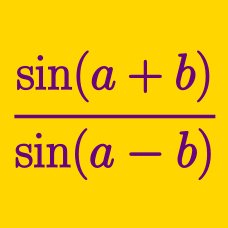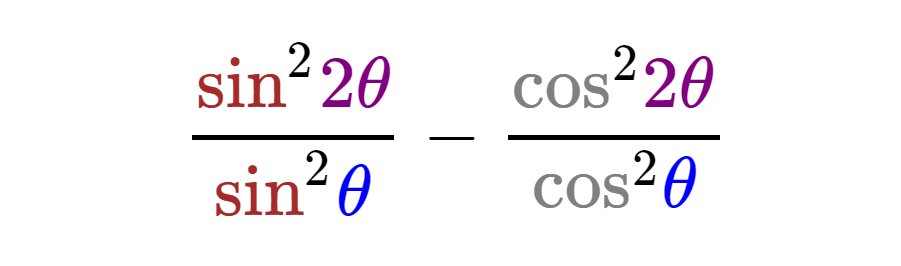Geometry

# Sum and Difference Trigonometric Formulas - Problem SolvingWhich of the following is equal to the above expression?

Rectangle $ABCD$ has side lengths $\overline{AB}=a, \overline{BC}=24.$ If point $P$ on $BC$ internally divides it $2:1$ and $\tan (\angle APD)=3$, what is the value of $a$?

If $\sin x=-\frac{3}{11}$, the value of $\frac{1-\cot x}{1+\tan x}+\frac{1+\cot x}{1-\tan x}$ can be expressed as $\frac{a}{b}$, where $a$ and $b$ are corpime positive integers. What is $a+b$?

If $\tan \theta + \cot \theta = 16$, what is the value of $\frac{\csc \theta}{\sec \theta - \tan \theta} + \frac{\csc \theta}{\sec \theta + \tan \theta}$?

Details and assumptions

Clarification: The function csc, is also known as cosec.

If $\sin \theta+\cos \theta=\frac{1}{6}$, the value of $\frac{\csc \theta}{\sec \theta-\tan \theta}+\frac{\csc \theta}{\sec \theta+\tan \theta}$ can be expressed as $-\frac{a}{b}$, where $a$ and $b$ are copime positive integers. What is $a+b$?

×